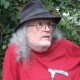## Time, Topology, Differential Logic • 5

And the founder, having shod a plough with a brazen ploughshare, and having yoked to it a bull and a cow, himself drove a deep furrow round the boundary lines, while those who followed after him had to turn the clods, which the plough threw up, inwards towards the city, and suffer no clod to lie turned outwards.

Plutarch • Life of Romulus

Re: Peirce List Discussion • ETETJBDJAJA

Our inquiry now calls on the rudiments of topology, for which I turn to J.L. Kelley.

### Chapter 1. Topological Spaces

#### 1.1. Topologies and Neighborhoods

A topology is a family$\mathcal{T}$ of sets which satisfies the two conditions:  the intersection of any two members of$\mathcal{T}$ is a member of$\mathcal{T},$ and the union of the members of each subfamily of$\mathcal{T}$ is a member of$\mathcal{T}.$  The set$X = \bigcup \{ U : U \in \mathcal{T} \}$ is necessarily a member of$\mathcal{T}$ because$\mathcal{T}$ is a subfamily of itself, and every member of$\mathcal{T}$ is a subset of${X}.$  The set${X}$ is called the space of the topology$\mathcal{T}$ and$\mathcal{T}$ is a topology for${X}.$  The pair$(X, \mathcal{T})$ is a topological space.  When no confusion seems possible we may forget to mention the topology and write “${X}$ is a topological space.”  We shall be explicit in cases where precision is necessary (for example if we are considering two different topologies for the same set${X}$).

The members of the topology$\mathcal{T}$ are called open relative to$\mathcal{T},$ or$\mathcal{T}$-open, or if only one topology is under consideration, simply open sets.  The space${X}$ of the topology is always open, and the void set is always open because it is the union of the members of the void family.  These may be the only open sets, for the family whose only members are${X}$ and the void set is a topology for${X}.$  This is not a very interesting topology, but it occurs frequently enough to deserve a name;  it is called the indiscrete (or trivial) topology for${X},$ and$(X, \mathcal{T})$ is then an indiscrete topological space.  At the other extreme is the family of all subsets of${X},$ which is the discrete topology for${X}$ (then$(X, \mathcal{T})$ is a discrete topological space).  If$\mathcal{T}$ is the discrete topology, then every subset of the space is open.  (Kelley, p. 37).

### References

• Kelley, J.L. (1955), General Topology, Van Nostrand Reinhold, New York, NY.
• Plutarch, “Romulus”, in Plutarch’s Lives : Volume 1, Bernadotte Perrin (trans.), Loeb Classical Library, William Heinemann, London, UK, 1914.

### 5 Responses to Time, Topology, Differential Logic • 5

1.Gary Herstein says: